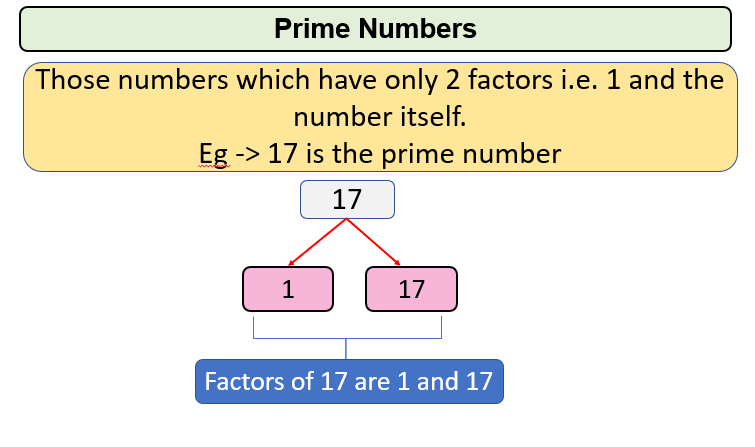# Prime Numbers in a given range

Problem Statement: Given a and b, find prime numbers in a given range [a,b], (a and b are included here).

Examples:

```Examples:
Input: 2 10
Output: 2 3 5 7
Explanation: Prime Numbers b/w 2 and 10 are 2,3,5 and 7.

Example 2:
Input: 10 16
Output: 11 13
Explanation: Prime Numbers b/w 10 and 16 are 11 and 13.
```

### Solution

Disclaimer: Don’t jump directly to the solution, try it out yourself first.Approach: Prime numbers b/w a and b can be found out by iterating through every number from a and b and checking for the number whether it is a prime number or not.

For E.g.

a=10

b=18

Let’s begin from 10

1. 10 is not prime.
2. 11 is prime.
3. 12 is not prime.
4. 13 is prime.
5. 14 is not prime.
6. 15 is not prime
7. 16 is not prime.
8. 17 is prime.
9. 18 is not prime.

So, print 11, 13, and 17 as prime numbers.

Code:

## C++ Code

``````#include <iostream>
#include <math.h>
using namespace std;
bool checkprime(int num)
{
if (num == 1)
return false;
int i = 2;
for (i = 2; i < sqrt(num); i++)
{
if (num % i == 0)
return false;
}
return true;
}
void PrintPrimesbwrange(int a, int b)
{
for (int i = a; i <= b; i++)
{
if (checkprime(i))
{
cout << i << " ";
}
}
}
int main()
{
int a = 11, b = 17;
PrintPrimesbwrange(a, b);
return 0;
}
``````

Output: 11 13 17

Time Complexity: O(N2) Since two nested loops are used.

Space Complexity: O(1)

## Java Code

``````public class Main {
public static boolean isPrime(int num) {
if (num == 1)
return false;
for (int i = 2; i < Math.sqrt(num); i++) {
if (num % i == 0)
return false;
}
return true;
}
public static void PrintPrimesbwrange(int a, int b) {
for (int i = a; i <= b; i++) {
if (isPrime(i)) {
System.out.print(i + " ");
}
}
}
public static void main(String args[]) {
int a = 10, b = 17;
PrintPrimesbwrange(a, b);
}
}
``````

Output: 11 13 17

Time Complexity: O(N2) Since two nested loops are used.

Space Complexity: O(1)

Special thanks to Gurmeet Singh for contributing to this article on takeUforward. If you also wish to share your knowledge with the takeUforward fam, please check out this article